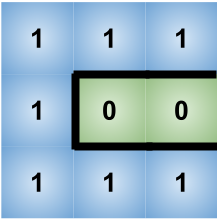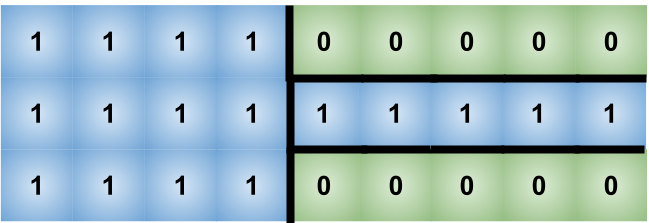0

# Contain Virus

Difficulty: HARD
Avg. time to solve
45 min
Success Rate
55%

Problem Statement
Suggest Edit

#### Given the state of each cell, your task is to find the number of walls you used to stop as many cells as possible from being infected.

##### Input Format:
``````The first line contains an integer ‘T’ which denotes the number of test cases or queries to be run. Then the test cases are as follows.

The first line of each test case contains two space-separated integers ‘N’ and ‘M’, denoting the dimensions of the country model.
Each of the next ‘N’ lines contain ‘M’ elements each denoting the state of the cell.
``````
##### Output Format:
``````For each test case, print a single integer denoting the number of walls required.

Print the output of each test case in a separate line.
``````
##### Note:
``````You don’t need to print anything; It has already been taken care of. Just implement the given function.
``````
##### Constraints:
``````1 <= T <= 10
1 <= N, M <= 100
0 <= X <= 1

Where ‘T’ is the number of test cases, ‘N’ and ‘M’ denotes the dimensions of the model, ‘X’ denotes the element of the matrix.

Time limit: 1 sec
``````
##### Sample Input 1:
``````2
3 3
1 1 1
1 0 0
1 1 1
3 9
1 1 1 0 0 0 0 0 0
1 0 1 0 1 1 1 1 1
1 1 1 0 0 0 0 0 0
``````
##### Sample Output 1:
``````5
13
``````
##### Explanation of sample input 1:
``````In the first test case, we can see in the above model, in order to save the uninfected cells from getting infected, we would require 5 walls.
````````````In the second test case, we can see in the model, in order to save the uninfected cells from getting infected, we would require 13 walls.
``````##### Sample Input 2:
``````2
2 2
1 0
0 0
3 3
1 1 1
1 0 1
1 1 1
``````
##### Sample Output 2:
``````2
4
``````Console